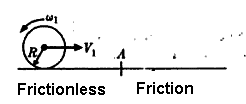# Angular momentum conservation.

Telemachus

## Homework Statement

Hi there. I have some doubts about an example I've found on the Ingard book of mechanics, matter and waves.

It says:
It is given to a homogeneous cylinder a horizontal speed V1 and an angular speed in opposed sense to that of the needles of the clock $$\omega_1=\frac{V_1}{R}$$ in the exempt part of frictions of a horizontal surface. But there of the point A, it changes the surface so that to the right of A the friction coefficient it is $$\mu$$Once it has happened of A the cylinder, will slip firstly on the rough plan, but it will finish rotating without slipping. In that point when it began to rotate without slipping which will the speed corresponding of the center of mass be?
The only force that it is exercised on the body in the address of the movement it's the contact force and in consequence, their action line is in the plane. Therefore, the angular moment of the cylinder regarding a point of reference of the plane, will remain constant during the whole movement.

I don't understand how its deduced that the angular momentum will be conservative respect a point over the plane. Could somebody help me to make this clear? The underline and the bold marks the principle and conclusion that I don't understand. How does it implies that the force is actioned over the plane the conservation of angular momentum?

Thanks!

## Answers and Replies

Homework Helper
The question whether there is a change in angular momentum reduces to the very simple question: is there any torque acting on the cylinder? Angular momentum (L) will be constant only if the torque (= rate of change of L) is 0: $\tau = dL/dt$

If I understand the question correctly, it appears to me that there is a torque on the cylinder while it slips on the frictional surface. T magnitude of L not only changes: its direction reverses.

AM

Telemachus
Yes, I think I got it. There is no torque because its making the considerations taking a point over the plane, which means r=0, and thats way angular momentum conserves for any point over the plane.

Thank you Andrew.